## 4.1.1 Maxwell's Equations

The basic equations solved in a device simulator can be derived from Maxwell's equations. The four partial differential equations relate the electric field (), the displacement field (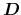), the magnetic field (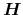), and the induction field (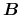) vectors to the current density (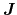) and the electric charge density (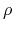):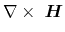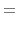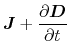(4.1)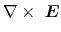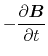(4.2)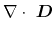(4.3)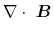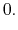(4.4)

S. Vitanov: Simulation of High Electron Mobility Transistors